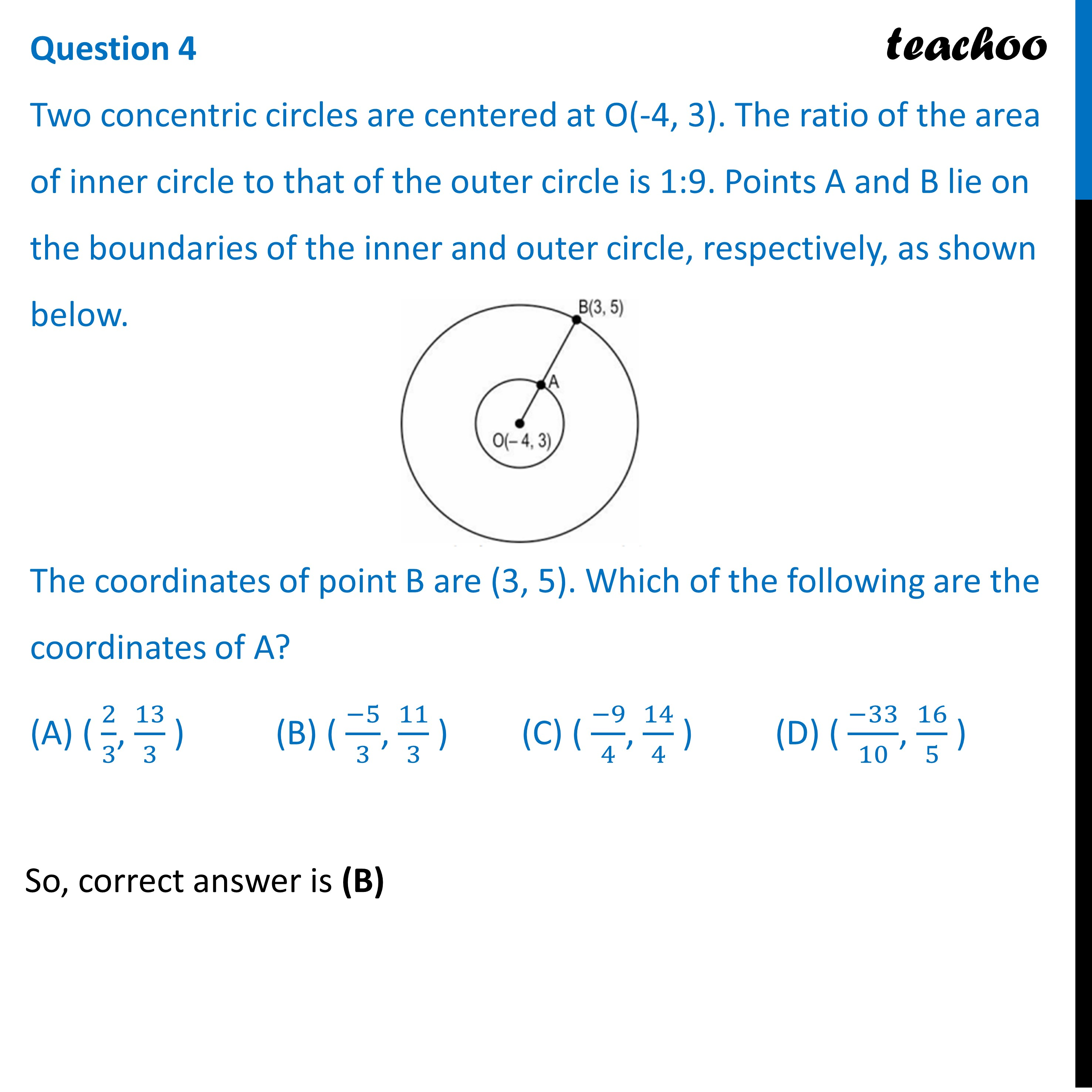Practice Questions CBSE - Maths Class 10 (2023 Boards)

Class 10
Solutions of Sample Papers for Class 10 Boards

## (D) ( (-33)/10, 16/5  )Learn in your speed, with individual attention - Teachoo Maths 1-on-1 Class

### Transcript

Two concentric circles are centered at O(-4, 3). The ratio of the area of inner circle to that of the outer circle is 1:9. Points A and B lie on the boundaries of the inner and outer circle, respectively, as shown below. The coordinates of point B are (3, 5). Which of the following are the coordinates of A? (A) ( 2/3, 13/3 ) (B) ( (−5)/3, 11/3 ) (C) ( (−9)/4, 14/4 ) (D) ( (−33)/10, 16/5 )